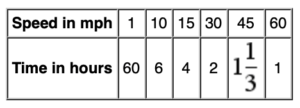# Inversely Proportional

A relationship between two quantities that has an inverse proportion means that as one quantity becomes smaller, the other becomes larger. An example of inversely proportional quantities is the relationship between the speed and time it takes to travel a fixed distance. If you drive 60 mph, you can drive 60 miles in 1 hour. If you drive 30 mph, it will take you 2 hours to drive the same 60 miles.Inversely proportional relationships have a constant of proportionality. It can be found from a combination of the speed and time that works for all pairs of speed and time. What is the constant of proportionality for the above relationship? How does the graph of this relationship relate to the graph of a proportional relationship? How long will it take you to drive 60 miles if you drive at 2 mph? 25 mph? 65 mph? How fast must you drive to cover the 60 miles in 5 hours? 3 hours?Extension:
Identify some real-world examples of inversely proportional relationships and graph some sample data. Determine what the general characteristics a graph of two inversely proportional quantities are.